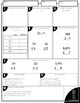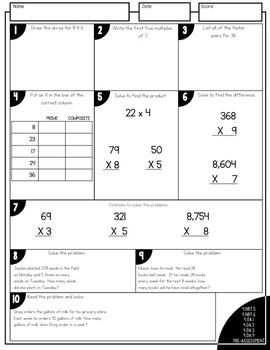# 4th Grade Guided Math - MultiplicationSubject
Resource Type
Format
PDF (17 MB|263 pages)
Standards
\$15.00

#### Also included in

1. Fourth Grade Guided Math: This 4th grade Guided Math Yearlong Curriculum Bundle incorporates EVERYTHING you will need to easily and effectively implement Guided Math in your 4th Grade classroom. Implementing Guided Math in fourth grade has never been easier! Check out this Guided Math curriculum bun
\$96.00
\$120.00
Save \$24.00

### Description

Fourth Grade Guided Math: This is the second unit in my 4th Grade Guided Math curriculum. This unit focuses on multiplication and includes EVERYTHING you will need to quickly and efficiently implement Guided Math in your 4th Grade classroom.

It is completely aligned to the Common Core State Standards.

This 4th Grade Guided Math resource includes 14 DIFFERENTIATED lessons on the following concepts:

* Meaning of multiplication
* properties of multiplication
* factors in multiplication
* multiples in multiplication
* distributive property of multiplication
* multiplication using bar models
* multiples of ten
* round to estimate products
* multiply a 2-digit number by a 1-digit number
* multiply a 2-digit number by a 1-digit number (with regrouping)
* multiply a 3-digit number by a 1-digit number (with regrouping)
* multiply a 4-digit number by a 1-digit number
* problem solving using multiplication
* multiply a 2-digit number by a 2-digit number

This 4th Grade Guided Math Multiplication Resource includes:

☞ Fully Detailed and Differentiated Lesson Plans for Small Group Instruction

☞ Information Guide on HOW TO effectively implement Guided
Math in your classroom (images and text instructions)

☞ Independent Practice Printables / Interactive Notebook activities
aligned to each small group instruction lesson

☞ Guided Practice Workmats for EACH lesson to help guide your
small group instruction

☞ 20 Word Problems for Math Journals

☞ 15 Math Stations - 5 SPIN-ITS Math Stations, 5 Roll-Its Math Stations, and 5 Solve-Its Math Stations

☞ Pre and Post Assessments on numeracy and operations

☞ Guided Math Organization tools - Guided Math Binder Cover,
Binder Spines, Binder dividers lesson plan templates, anecdotal
notes template, weekly lesson plan template, math journal labels,
math station labels, large poster-sized iChart (print at office
supply store), math station recording sheet, and fact fluency
station labels

☞ Links to resources and tools that I use during my Guided Math
instruction

This Guided Math Numeracy and Operations Resource is JAM-PACKED with EVERYTHING you need to quickly and efficiently implement Guided Math in your 4th Grade Classroom!

If you are interested in this resource, please check out the BUNDLE to save a BUNDLE!

Total Pages
263 pages
N/A
Teaching Duration
3 Weeks
Report this Resource to TpT
Reported resources will be reviewed by our team. Report this resource to let us know if this resource violates TpT’s content guidelines.

### Standards

to see state-specific standards (only available in the US).
Find all factor pairs for a whole number in the range 1-100. Recognize that a whole number is a multiple of each of its factors. Determine whether a given whole number in the range 1-100 is a multiple of a given one-digit number. Determine whether a given whole number in the range 1-100 is prime or composite.
Solve multistep word problems posed with whole numbers and having whole-number answers using the four operations, including problems in which remainders must be interpreted. Represent these problems using equations with a letter standing for the unknown quantity. Assess the reasonableness of answers using mental computation and estimation strategies including rounding.
Multiply or divide to solve word problems involving multiplicative comparison, e.g., by using drawings and equations with a symbol for the unknown number to represent the problem, distinguishing multiplicative comparison from additive comparison.
Interpret a multiplication equation as a comparison, e.g., interpret 35 = 5 × 7 as a statement that 35 is 5 times as many as 7 and 7 times as many as 5. Represent verbal statements of multiplicative comparisons as multiplication equations.
Find whole-number quotients and remainders with up to four-digit dividends and one-digit divisors, using strategies based on place value, the properties of operations, and/or the relationship between multiplication and division. Illustrate and explain the calculation by using equations, rectangular arrays, and/or area models.

### Q & A

Teachers Pay Teachers is an online marketplace where teachers buy and sell original educational materials.